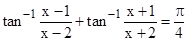Request a call back

Consider the equation. Choose the CORRECT option(s). The sum of all solutions = 0< The number of solutions = 2< The number of solution = 1< The sum of squares of all solutions = 1<
Asked by harshsharma2531 | 09 Aug, 2020, 09:28: AMExpert Answer
To find solutions of the equation tan-1(x-1/x-2) + tan-1(x+1/x+2) = pi/4Answered by Renu Varma | 09 Aug, 2020, 04:32: PM

## Concept Videos

CBSE 12-science - Maths
Asked by joymaibam38 | 26 Dec, 2021, 09:04: AMANSWERED BY EXPERT
CBSE 12-science - Maths
Asked by naikmanjuank | 17 Sep, 2020, 11:48: AMANSWERED BY EXPERT
CBSE 12-science - Maths
Asked by sshas3199 | 23 Aug, 2020, 09:24: PMANSWERED BY EXPERT
CBSE 12-science - Maths
Asked by raiannu064 | 16 Jan, 2020, 01:03: PMANSWERED BY EXPERT
CBSE 12-science - Maths
Plz solve (x) part Find the value of xAsked by lovemaan5500 | 04 Jan, 2020, 11:12: PMANSWERED BY EXPERT
CBSE 12-science - Maths
Asked by patelmanan4102002 | 05 Aug, 2019, 08:21: PMANSWERED BY EXPERT
CBSE 12-science - Maths
Asked by mridulabarua05 | 21 Feb, 2019, 01:05: PMANSWERED BY EXPERT
CBSE 12-science - Maths
Asked by Suraj2000reddy5 | 27 Jan, 2019, 10:08: PMANSWERED BY EXPERT
CBSE 12-science - Maths
Asked by niharikapabba2605 | 11 Jun, 2018, 06:22: PMANSWERED BY EXPERT